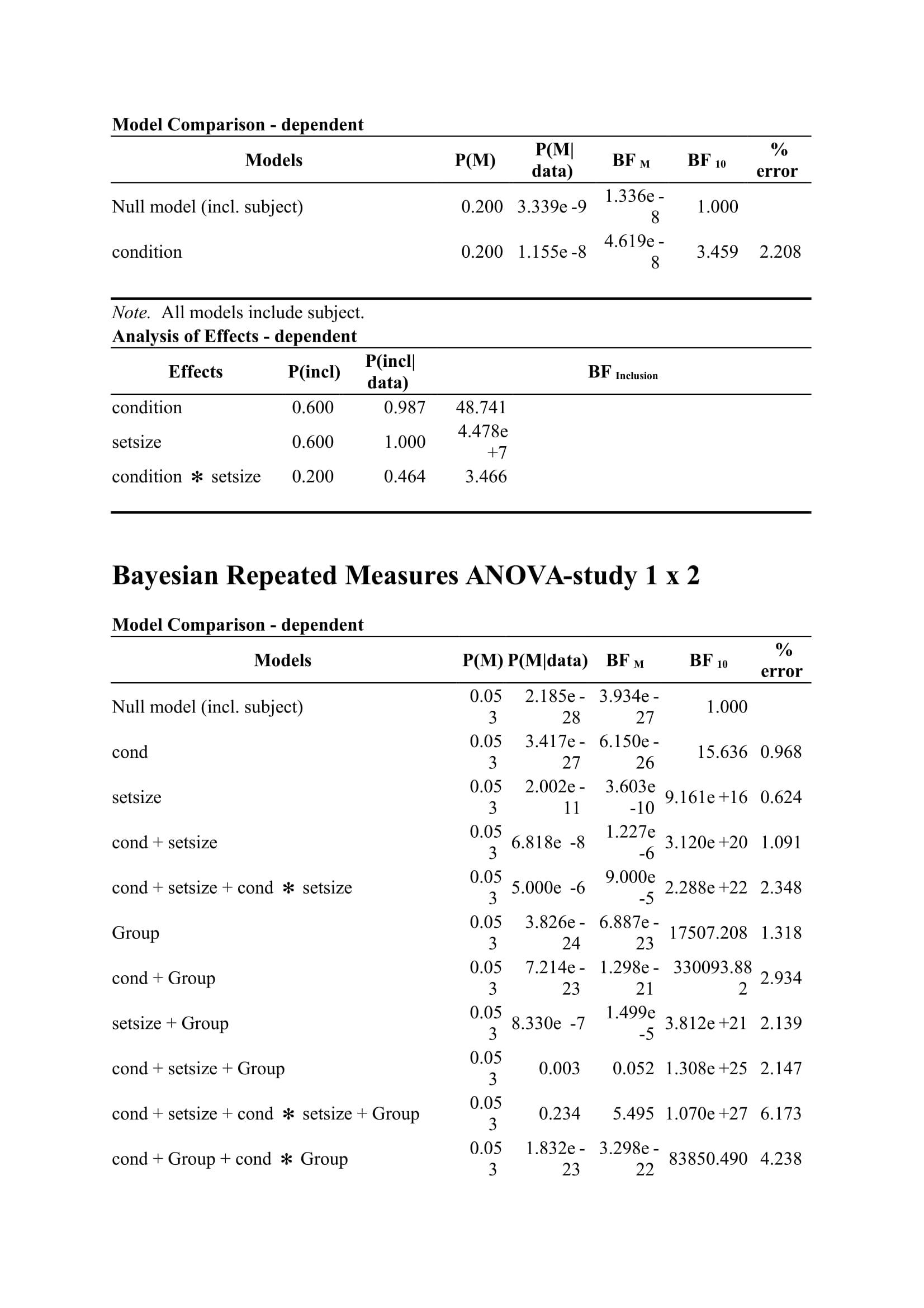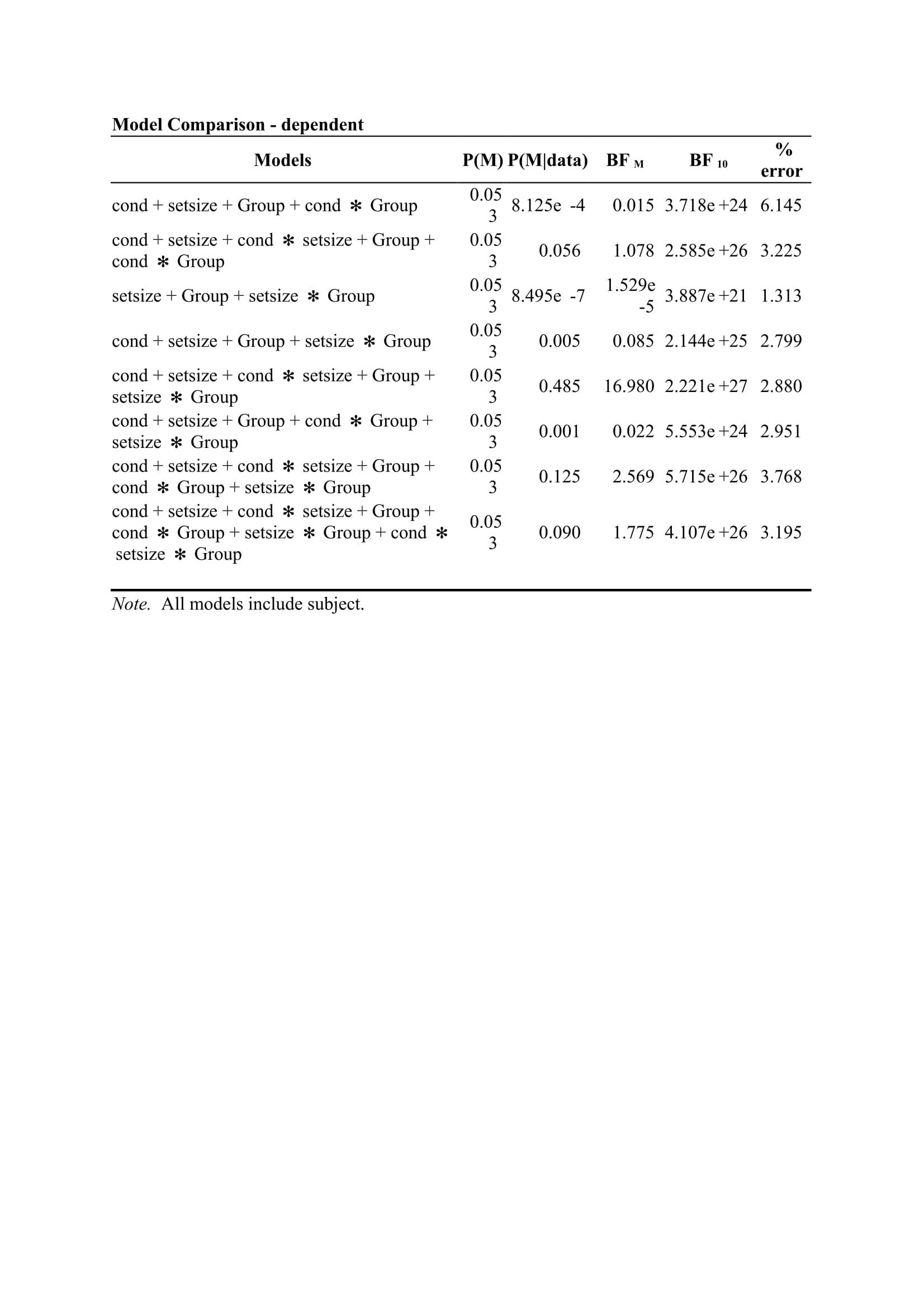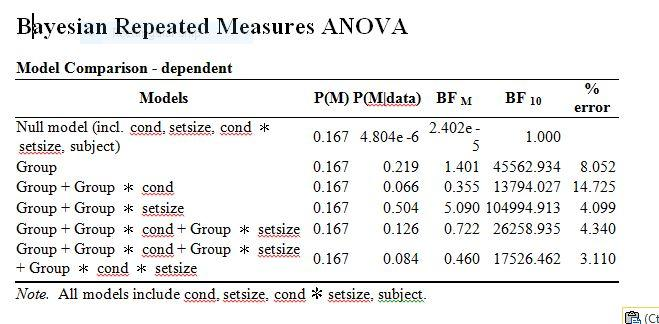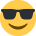#### Howdy, Stranger!

It looks like you're new here. If you want to get involved, click one of these buttons!

Supported by

# Bayesian repeated measure ANOVA

Good morning,

A reviewer is asking us to confirm that our null results (i.e., no main effect of group and no group x RG interaction) highlighted trough a regular ANOVA support, or not, the null hypothesis.
We therefore decided to apply Bayesian statistics on the data with JASP. Here is a table of the results.

Would it be correct in this specific case to mention that: "According to Bayesian statistics conducted with JASP, the absence of difference involving the group factor can only be taken as anecdotal evidence given that none of the fitted models are substantially better than the null model (1/3 < all BF10 < 1)"?

Indeed we find that:

• Group vs. null model = 0.805/1 = 0.805 = Anecdotal evidence for H0
• Interaction model vs. null = 0.607/1 = 0.607 = Anecdotal evidence for H0

Would there be anything else to report?

A--

• Dear A,
Yes, I think that's spot-on. I would report the results you mention, and I would also put an annotated .jasp file on the Open Science Framework. If you provide the link then everybody can view the output even without having JASP installed.
Cheers,
E.J.

• Hi everyone,
I recently started using the JASP software. I am conducted a Bayesian RM ANOVA for my data and obtained the output attached. My questions are:
1. Given the data, is there a main effect of setsize? If there is, is it in support of alternative or null model. What is the BF for that? Is the evidence anecdotal, marginal or strong?
2. Given the data, is there a main effect of condition? If there is, is it supportive of alternative or null model? What is the BF for that? Is the evidence anecdotal, marginal or strong?
3. Is there evidence for interaction between the two: condition x setsize? If there is, how can I show this from the result? In other words, what is the BF for the interaction? Is the evidence anecdotal, marginal or strong?
4. Is there a main effect of group? If there is, is this evidence in support of alternative or null model?
5. Is there condition x setsize x group interaction? If there is, is this evidence in support of alternative or null?

I have labelled the outputs for ease of understanding. The first output is for study 1. The second output is for study 2. The third output is comparing the two studies (study 1 and study 2).

Tom• Hi Tom,

We work through an example in part II of a series available here: https://osf.io/m6bi8/

Cheers,
E.J.

• Thanks EJ for the link. I will check it out.

• Hi EJ,

Thanks once again for all your quick response to my queries and offering excellent support. I have looked at the paper you recommended and have now come up with the following conclusions with regards to my data. Please kindly let me know if I'm on the right track. Ok, here it goes:

1. To get the main effect of condition, I dividing the BF10 of the model with both main effects by the BF10 of the model with the main effect of factor 2 to determine the main effect of factor 1 (condition). In my output, this yielded 9.154e10/3.189e9 = 3.189. I then concluded that the data were 3.19 times more likely under the alternate hypothesis of an effect of condition than the null hypothesis predicting no effect of condition. The data provide moderate evidence for the hypothesis on the effect of condition.

2. To get the main effect of set size, I dividing the BF10 of the model with both main effects by the BF10 of the model with the main effect of factor 1 to determine the main effect of factor 2 (set size). In my output, this yielded 9.154e10/1.794 = 51. I then concluded that the alternative hypothesis was 51 times more likely than the null hypothesis for the effect of set size. The data provide very strong evidence for the hypothesis on the effect of set size.

3. To get the interaction, I dividing the BF10 of the full model with both main effects and interaction effect by the BF10 of the model with both main effects to determine the interaction effect. In my output, this yielded 9.154e10/2.936e12 = 2.57. I then concluded that the alternative hypothesis was 2.6 times more likely under the two main effects model than the null hypothesis when the model for interaction is added. This provides anecdotal evidence in support of the hypothesis that condition and set size interact on accuracy

4. I also looked at the effects option, which computes the factor inclusion probabilities by averaging across the models. As can be seen, there is overwhelming evidence for including the interaction, yielding a Bayes factor of 124. I also mentioned that the reason for the discrepancy (i.e., a Bayes factor of 2.6 against the interaction model versus a Bayes factor of 124 in favour of the interaction model) is because the two are addressing different question. Importantly, the BF of 2.6 compares the full model against a pretty good alternative (i.e., the two main effect models); in the case of the BF = 124, all candidate models are considered including the ones that happen not to do well. Overall, this suggests that the two main effects model has predicted the data relatively poorly compared to the null model or one of the single main effects models.
5. Looking at items 1-4 above, have I left something out? Is there anything else I need to talk about?

***Please note the above interpretation was used for study 1.

In terms of interpreting the JASP output for study 1 and 2 (mixed-ANOVA effect), I'm really stuck in terms of the formula to use in the analysis/for the interpretation. I want to find out if:

a) there is main effect of group
b) there is interaction between group and set size. If yes, how do I determine this?
c) there is interaction between group and condition. If yes, how do I determine this?

Thank you in advance for your continuous support. I also thank the rest of the JASP team for all their effort and generous package.

Regards,

Tom

• Hi Tom,

Let's look at the interaction first. The two main effects model has BF10 = 9.154e10. Adding the interaction makes the model much stronger (compares to the null): BF10 = 2.936e12. So the evidence for including the interaction is 2.936e12/9.154e10 = 32. I think when you divided you forgot to deal with the e10 and e12.

So however way you look at this, there is good evidence for the interaction. There are various ways to look at the importance of the main effects, but it seems to me that all of them matter.

Cheers,
E.J.

• Hi E.J., many thanks for your response. But I'm completely lost with couple of things.

First, how do you arrive at 32. When I performed the similar calculation, I obtained the following:
2.936e+12 = 35.232 (2.936 x 10^12)
9.154e+10 = 91.54 (9.154 x 10^10)
35.232/91.54 = 0.38

When you said I forgot to deal with e10 and e12, please I don't get it. Kindly clarify.

Second, in the transitive formula for obtaining interaction, what is the numerator in the formula? Is it the model with the two main effects or the model with the two main effect plus interaction? Your previous examples to some of the posts I've read suggest so, including the examples in your paper "Bayesian inference for Psychology. Part 11: Example Applications with JASP". In that paper you always have the model with the two main effect as the numerator. If that is the case, then it is supposed to be 9.154e10/2.936e12 instead of 2.936e12/9.154e10. Is there something I'm not getting here? Please kindly advise.

Thanks once again.

Cheers,
Tom

• Hi Tom,

It's my turn to be lost. We have 2.936 x 10^12 for the full model and 9.154 x 10^10 for the main effects only model. This means that the support for the full model over the main effects only model is (2.936 x 10^12) / (9.154 x 10^10) = (2.936 x 10^2) / 9.154 = 32.

In the formula for the transitivity, it does not really matter what the numerator or denominator is, as long as the term that relates to the null hypothesis drops out.

Cheers,
E.J.

• Many thanks E.J. for breaking this down to me. I now see where I made mistake. Indeed, I forgot to deal with the e10 and e12. However, as you said that it doesn't matter about the numerator or denominator, if I swap it round 9.154/(2.936x10^2) it will give me 0.03. How do I then interpret the result since obviously they yielded different values? Recall the other value was 32.

Also, please can you advise if the formula I used for the main effect above is correct? For instance, to get the main effect of condition (Factor 1), I divided the BF10 of the model with both main effects by the BF10 of the model with the main effect of factor 2 to determine the main effect of factor 1 (condition). In my output, this yielded 9.154e10/3.189e9 = 2.87. I then concluded that the data were 2.87 times more likely under the alternate hypothesis of an effect of condition than the null hypothesis predicting no effect of condition. The data provide moderate evidence for the hypothesis on the effect of condition. Please is this correct?

Lastly, how do I calculate (a) the main effect of group (b) group x set size interaction (c) group x condition interaction???

Regards,

Tom

• Hi Tom.

Sorry for the tardy reply. Here are some remarks that I hope will help:
1. You can either say the BF is 32 in favor of model A versus model B, or you can say that the BF is 1/32 = 0.03 in favor of model B versus model A. These statements are equivalent.

1. One way to determine whether there is an effect of condition is to look at the model with setsize (which beats the null with a BF of 3.189e9) and then see what happens if you add condition; this model beats the null more decisively, with a BF of 9.154e10. If you divide these, you get 9.154e10/3.189e9 = 28.70 (! note the change in the "e" factor). So the data are almost 30 times more likely under the two factor model than under the model with only setsize. But in general I am in favor of reporting and discussing the entire table.

Cheers,
E.J.

• Hi E.J.,

Sincere apologies for my late reply. I've been away for weeks. Thanks for your response to my question. "So the data are almost 30 times more likely under the two factor model than under the model with only setsize"- please how do I interpret this in relation to the main effect of condition?

Secondly, I guess I'll follow the same rule when looking at the main effect of setsize. In that case, I will look at the model with condition (which I think also beats the null with a BF of 1.794. So I will have to divide 9.154e10/1.794. Is that right?

Lastly, I want to know if there is main effect of group (i.e., Experiment 1 vs Experiment 2) and also whether there is interaction between group and set size on the one hand, and group and condition on the other. Sorry I kept asking this question as I'm not sure how this is interpreted.

Thanks once again for your guidance and support.

Regards,

Tom

• Hi Tom,

1. " "So the data are almost 30 times more likely under the two factor model than under the model with only setsize"- please how do I interpret this in relation to the main effect of condition?"

I am not sure what you want to know, exactly. What I see is that in Experiment 1, you have good evidence for the presence of all factors, including the interaction. In Experiment 2, the pattern is the same except that you don't have evidence for including the interaction.

1. Correct.

2. For an overall assessment of the effect of group, I would turn to an "analysis of effects", that averages across the models involved. For the assessment of your interactions, you have to think about which models you want to compare, and then divide the BFs. The pattern may be easier to interpret of you add "condition", "set size", and "condition * set size" as nuisance variables, so that they are always in the model. Alternatively, you can look at an analysis of effects here as well.

Cheers,
E.J.

• Many thanks once again E.J. for your response. In terms of the first question, it is all clear and your interpretation in terms of Experiment 1 and 2 is in line with what I found, which is good. For the second part, I will follow the guidelines suggested. Please I will get back to you if I'm stuck, hopefully not. In the meantime, I'll give it a go as suggested.

Thanks once again for your kind and generous response. Much appreciated.

Take care.

Cheers,

Tom

•Hi E.J.,

Just to thank you once again for all your support with my analysis and interpretation. I have finished my analysis for Experiments 1 and 2. However, I need few guidance with respect to the comparison between Experiments 1 and 2, which in traditional ANOVA is called "Mixed Anova". I am interested in knowing the following:

A) Main effect of group(experiment): Is performance in Experiment 1 better than Experiment 2?Group x set size interaction: Is there an interaction between group and set size? In other words, is there a set size effect in both experiments? If there is, as independent analysis of each experiment reveals there is, I expect evidence in favour of the null hypothesis than the alternate hypothesis
C) Group x condition interaction: Is there an interaction between group and condition? In other words, is there an effect of condition in both experiments? If there is, as independent analysis of each experiment reveals there is, I expect evidence in favour of the null hypothesis than the alternate hypothesis

You suggested I do the following:

1. "For an overall assessment of the effect of group, I would turn to an "analysis of effects", that averages across the models involved". Please which "analysis of effects" are you referring to?
2. For the assessment of your interactions, you have to think about which models you want to compare, and then divide the BFs. The pattern may be easier to interpret of you add "condition", "set size", and "condition * set size" as nuisance variables, so that they are always in the model.

In response to your suggestions, I have added "condition", "set size", and "condition * set size" as nuisance variables, so that they are always in the model (see the output attached). Then I did the following:

a) Main effect of group: looking at the output, this yields a BF= 45562.934. I'm not sure if this is enough or whether I need to apply the transitive formula here. If that is the case, which BF do I need to divide this BF with to obtain the main effect of group?

b) Group x set size interaction: I compared the model containing 'Group x condition' to the model containing 'Group + Group  ✻  cond + Group  ✻  setsize'. So I obtained 24468.725/11487.243 = 2.13

c) Group x condition interaction: I compared the model containing 'Group x set size' to the model containing 'Group + Group  ✻  cond + Group  ✻  setsize'. So I obtained 24468.725/95244.322 = 0.25

Please kindly advice in respect to the above. Sorry for being a pain, but this seems to be the last part of my analysis of which I'm still not clear of what to do.

Cheers,

Tom

• Hi Tom,

a) Yes there is a clear effect of group. No transitivity calculation needed
b & c) for the interaction effects, I would note that the best supported model is the one that has both group and the group * set size interaction. BTW, I don't see the numbers 24468.725 and 11487.243 in the output. But it is clear what the model with the group*set size interaction will have a BF of about 2 versus the model with only group. For all other models, the interaction models are not supported by the data (the model with only group receives more support).

Cheers,
E.J.

• Hi E.J.,

Thank you so much for your response. Sorry for the miss up with the numbers. Ok, here is the calculation:

a) Group x set size interaction: I compared the model containing 'Group + Group  ✻  cond' to the model containing 'Group + Group  ✻  cond + Group  ✻  setsize'. So I obtained 26258.935/13794.027 = 1.9

b) Group x condition interaction: I compared the model containing 'Group + Group  ✻  setsize' to the model containing 'Group + Group  ✻  cond + Group  ✻  setsize'. So I obtained 26258.935/104994.913 = 0.25

So as you said, the model with good support are the one containing group and group * setsize interaction with the former receiving more support. Please is this correct?

Thanks and best wishes for the rest of the day.

Cheers,

Tom

• Hi Tom,

Well, that's one way of doing it. You could also do comparisons to the simpler models, for instance instead of (a) you could compare "Group" to "Group x set size". If you want to average across all such possibilities you can report the analysis of effects. But basically you could report the entire table and talk the reader through it.

E.J.

• Hi E.J.,

Thank you so much for all your useful guidance and great support. Indeed, I have learnt a great deal chatting with you on this forum. I can now finish up my analysis and write up in peace. Please one last thing, you know the '% error' on the last column, do I need to report them as well? And what do they actually represent and how are they interpreted if I may ask?

Thanks and have a wonderful weekend.

Tom

• Sometimes the BF is determined by numerical techniques (MCMC sampling, or numerical integration). These techniques come with uncertainty, and this is what the %error indicates. As a rule of thumb, I would start to worry when it exceeds about 5%, and I would not report it in the main MS. You could copy-paste the entire JASP table and present it in an online appendix, however.

Cheers,
E.J.

• Thanks E.J. for explaining this. Much appreciated.

Cheers,

Tom

• Hello,

I've tried to analyse these results from what I understood in this forum and I would like to have a confirmation about what I got:

(See the upper table for my results)

1) The main effect of the "phase" condition: 156618.344/365559.172 = 0.428 --> 0.428 evidence for H1.

2) The main effect of the "aware" condition: 156618.344/0.327 = 478955.2 --> 478955.2 évidences for H1

3) The interaction effect: 98438.782/156618.344 = 0.629 --> 0.629 evidence for an interaction between "phase" and "aware".

That would be very helpful to tell me if I did it right.

I also encountered a little problem: I did the exact same test in the lower table, but JASP keep giving me slightly different results. What can I do about it?

Best,

Clément

• Dear Clement

Sorry for the tardy reply. In general, you can obtain evidence for any two models using transitivity, the way you outlined. But there are two crucial steps, one that comes before and one that comes after:

1. The step before: which models do you want to compare, exactly? There is no hard and fast rule here. I personally like the "analysis of effect" method (which averages across all models), and especially the "across matched models" functionality, but opinion differs. You did not outline exactly what models you would like to compare.
2. The step after: if you say, for instance: "The interaction effect: 98438.782/156618.344 = 0.629 --> 0.629 evidence for an interaction between "phase" and "aware" then I hope we agree that the interaction is supported *less* well than the two-main effect model.

Finally, you get slightly different answers because the result is not analytic but there is a numerical approximation. You can increase the number of samples (under Advanced, I think) to decrease the error, at the cost of the program running longer. You will never entirely get rid of it, however.

Cheers,

E.J.

• Dear E.J., may I ask you a question about my results?

My experiment have 3 independent variables, group, codi and other-self.

The model I am interested(my hypothese) in is the model included group, codi, other-self and 4 interactions(group*codi, group*other-self, codi*other-self and the interaction of these 3 variables).

However, the interested model here has a BF of 2.246e+56, it is far smaller than the best model(codi, group and their interaction).

In this situation, how can I interpret my result? Can I say that my hypothese is supported by the data, for its larger than the null model? Or I should take the best model(codi, group and their interaction) as my result?

Best,

JASPlearner

• Hi JASPLearner,

I would take the best model as a point of departure. Comparisons to the null model are rarely informative.

Cheers,

E.J.